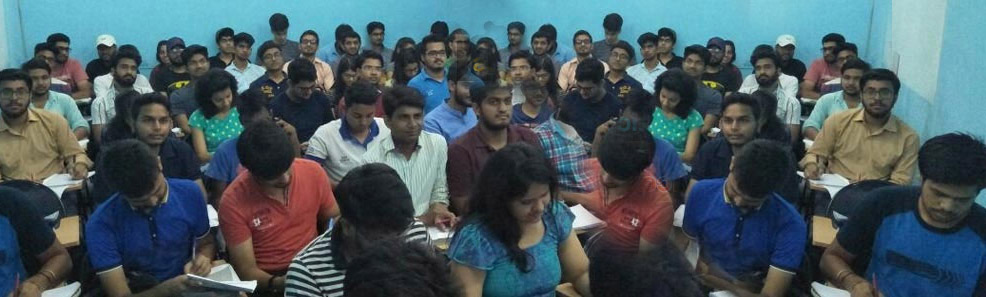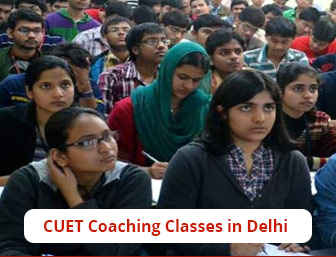## Computer Science and Information Technology Coaching Classes# Deep Institute - Computer Science and Information Technology (SCQP09)Deep Institute is an Entrenched, one-of-a-kind coaching genre, committed to offering Top-notch Comprehensive Training for the CUET exam. We are the Leading Computer Science and Information Technology Coaching Classes, as we upskill Students for Common University Entrance Test (CUET) which is a Common Platform for Candidates across the Country. Our institution develops students to be fully equipped to qualify for the CUET exam. We have an Exhaustive List of CUET-certified Students who have passed out with flying colors. We are acclaimed as the Best CUET Coaching in Delhi. Because we Engage Diligently with our Students, bringing in Remarkable Changes in their Conviction Levels & Life Skills, they have made it, with their incredible Presence Across the country.

Our Rigorous Curriculum is made to give students a Solid Grasp on the underlying ideas, and methods that sustain and support Computer Science and Information Technology Exams. We are the Most Popular & Preferred Coaching Classes for SCQP09. We are the Best Coaching for CUET in Delhi for the Preparations. Get enrolled today with CUET Coaching in Delhi and start brace yourself for the exam with us. We have exceptionally competent teachers to assist the students at all times.

### NOTE:

• There will be one Question Paper which will have 100 Questions.
• All questions will be compulsory.
• The Question Paper will have two parts i.e Part A and Part B.
• Part A will have 25 Questions based on Language Comprehension/Verbal Ability, General Awareness, Mathematics/Quantitative Ability, and Analytical Skills.
• Part B will have 75 questions based on Subject-Specific knowledge.

### Syllabus

Thinking and decision making: Creative thinking, unfamiliar relations, verbal reasoning, finding patterns trends, and assessment of figures & diagrams.

• Geometrical Designs & Identification
• Geometrical Designs & Identification
• Geometrical Designs & Identification
• Selection of related letters/words/numbers/ figures
• Identification of an odd thing/ item out from a group
• Completion of numerical series based on the pattern/logic
• Fill in the blanks of the series based on the numerical pattern and logic of the series
• Syllogism(Logis-based questions), Identification of logic & selection of correct answer based on the logic.

### Mathematics:

• Set Theory: Concept of sets - Union, Intersection, cardinality, Elementary counting; Permutations, and combination.
• Probability and statistics: Basic concepts of probability theory, Averages, dependent and independent events, frequency distribution, measures of central tendencies and dispersions.
• Algebra: Fundamental operations in algebra, expansion, factorization, simultaneous linear/quadratic equations indices, logarithms, arithmetic, geometric and harmonic progression, determinants, and matrices.
• Coordinate Geometry: Rectangular Cartesian coordinates, distance formulae, equation of a line, and intersection of lines, pair of straight lines, equation of a circle, parabola, ellipse, and hyperbola.
• Calculus: Limit of functions, continuous function, differentiation of function, tangents and normal, simple examples of maxima and minima. Integration of functions by parts, by substitution, and by partial fraction, definite integrals, applications of definite integrals to areas.
• Vectors: Position vector, addition and subtraction of vectors, scalar and vector products, and their applications to simple geometric problems and mechanics.
• Trigonometry: Simple identities, trigonometric equations, properties of triangles, solution of triangles, heights and distances, general solutions of trigonometric equations.

### Computer Awareness:

• Computer basics: Organization of a computer, Central Processing Unit, structure of instructions in CPU, input/output devices, computer memory and back-up devices.
• Data Representation: Representation Characters, integers and fractions, binary and hexadecimal representations, binary arithmetic: addition, subtraction, multiplication, division, simple arithmetic and two’s complement arithmetic, floating point representations of numbers, Boolean algebra, truth tables, Venn diagrams.